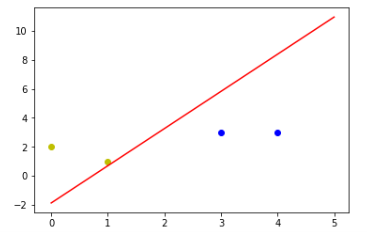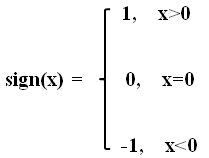# 机器学习——神经网络（一）：单层感知机

### 神经网络——单层感知机

python 3.4 、jupyter

import numpy as np
import matplotlib.pyplot as plt

# 载入数据（解释一下：x_data第一列为偏置值（bias），第二列和第三列就是输入数据;y_data即训练标签）
x_data = np.array([[1,3,3],
[1,4,3],
[1,1,1],
[1,0,2]])
y_data = np.array([,
,
[-1],
[-1]])
# 初始化设置权值（为了符合矩阵计算变为三行一列，取值范围为-0.5到0.5）
w = (np.random.random([3,1])-0.5)
print(w)
# 输出：
#[[-0.16266462]
#[ 0.02265906]
#[-0.22597695]]

# 设置学习率learning rate（这个学习率后面再重点提一下，现在正常设置一下就ok，但是设置不好得到的结果可能很不理想）
lr = 0.11
# 神经网络的输出（output）
O = 0
# .dot是矩阵乘法，而常规乘法是对应的元素相乘
def update():
'''更新函数，调用一次执行一次'''
global x_data,y_data,w,lr
O = np.sign(np.dot(x_data,w)) # np.sign函数时numpy中的取数字符号
w_c = lr*(x_data.T.dot(y_data-O))/int(x_data.shape)# 分母求得误差的总合，分子表示sample的数量，结果表示平均误差
w = w+w_c

for i in range(100):
update() # 权值更新
O = np.sign(np.dot(x_data,w)) # 计算当前输出
print("epoch:",i)
print("w:",w)
if(O==y_data).all():     # 如果当前输出与实际输出相当，模型收敛，循环结束（。all是全部相等的意思）
print("#####################")
print("finished")
print("epoch:",i)
print("#####################")
break
# 正样本
x1 = [3,4]
y1 = [3,3]
# 负样本
x2 = [1,0]
y2 = [1,2]

# 计算分界线的斜率以及截距
k = -w/w
b = -w/w
print("k = ",k)
print("b = ",b)

xdata = (0,5)
plt.figure()
plt.plot(xdata,xdata*k+b,'r')
plt.scatter(x1,y1,c='b')
plt.scatter(x2,y2,c='y')
plt.show()1. sign()函数1. 学习率 ilearning rate：控制模型的学习进度。更多请参考深度学习：学习率

Markdown小知识点：

• 使用html标签和markdown语句的嵌套也可以实现图片的居中操作等等
• 常规HTML使得图片居中<div align='center'><img src=''></div>
• 使用markdown的图片添加语句和HTML配合<span><div align=‘center’>[名字](地址)</div></span>09-30
10-2698409-207078
09-28551
08-0863
10-182188
04-061856
06-143042
01-145208
12-016908
05-312434